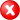#Boost C++ Libraries

...one of the most highly regarded and expertly designed C++ library projects in the world.

##### return_centroid

Calculates the centroid of a geometry.

###### Description

The free function centroid calculates the geometric center (or: center of mass) of a geometry. This version with the return_ prefix returns the centroid, and a template parameter must therefore be specified in the call..

###### Synopsis

```template<typename Point, typename Geometry>
Point return_centroid(Geometry const & geometry)```

###### Parameters

Type

Concept

Name

Description

Point

Any type fulfilling a Point Concept

-

Must be specified

Geometry const &

Any type fulfilling a Geometry Concept

geometry

A model of the specified concept

###### Returns

The calculated centroid

Either

`#include <boost/geometry.hpp>`

Or

`#include <boost/geometry/algorithms/centroid.hpp>`

###### Conformance

The function centroid implements function Centroid from the OGC Simple Feature Specification.

###### Behavior

Case

Behavior

Point

Returns the point itself as the centroid

Multi Point

Calculates centroid (based on average)

linear (e.g. linestring)

Calculates centroid (based on weighted length)

areal (e.g. polygon)

Calculates centroid

Empty (e.g. polygon without points)

Throws a centroid_exception

Cartesian

Implemented

Spherical

Calculates the centroid as if based on Cartesian coordinates

###### Supported geometries

2D

3D

PointSegmentBoxLinestringRingPolygonMultiPointMultiLinestringMultiPolygonLinear